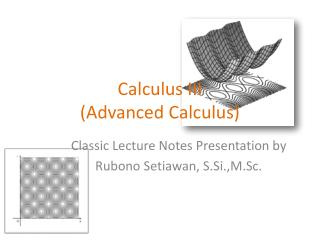Download PresentationCalculus III (Advanced Calculus) - PowerPoint PPT Presentation

Calculus III (Advanced Calculus). Classic Lecture Notes Presentation by Rubono Setiawan, S.Si.,M.Sc. Contents of Lesson. Vector and The Geometry of Space Differential Calculus of Function Differential Calculus of Function Multiple Integral Vector Calculus. Bibliography.I am the owner, or an agent authorized to act on behalf of the owner, of the copyrighted work described.
Download PresentationDownload Policy: Content on the Website is provided to you AS IS for your information and personal use and may not be sold / licensed / shared on other websites without getting consent from its author.While downloading, if for some reason you are not able to download a presentation, the publisher may have deleted the file from their server.

- - - - - - - - - - - - - - - - - - - - - - - - - - E N D - - - - - - - - - - - - - - - - - - - - - - - - - -
Presentation Transcript
1. Calculus III(Advanced Calculus) Classic Lecture Notes Presentation by Rubono Setiawan, S.Si.,M.Sc.

2. Contents of Lesson • Vector and The Geometry of Space • Differential Calculus of Function • Differential Calculus of Function • Multiple Integral • Vector Calculus Classic Lecture Notes of Calculus III by RubonoSetiawan, S.Si.,M.Sc.

3. Bibliography • E.J. Purcell, D. Varberg, Calculus Ninth Edition, Prentice Hall, Inc, New-York, USA, 1987. • J.Stewart, Calculus, Fifth Edition, ITP, Singapore. Classic Lecture Notes of Calculus III by Rubono Setiawan, S.Si.,M.Sc.

4. Contents of ‘’ KD 1 ‘’ • 1. Vector and The Geometry of Space • 2. Differential Calculus of Function • (Introduction, Function of Several Variables, Limit and Continuity ) Classic Lecture Notes of Calculus III by Rubono Setiawan, S.Si.,M.Sc.

5. Chapter 1. Vector and The Geometry of Space • First, Loot at the following figure below... • Wind Velocity is a vector because it has magnitude and direction. Picture are velocity vectors indicating the wind pattern over San Francisco Bay at 12 : 00 P.M on June 11, 2002 Classic Lecture Notes of Calculus III by Rubono Setiawan, S.Si.,M.Sc.

6. and then see also this following picture Classic Lecture Notes of Calculus III by Rubono Setiawan, S.Si.,M.Sc.

7. 1.1. Three Dimensional Coordinate Systems • In this chapter we introduce vectors and coordinate systems for three dimensional space. This material is important because this will be setting for our study of the calculus of functions of two variables in the next chapter and the graph of such function is a surface in space. Classic Lecture Notes of Calculus III by Rubono Setiawan, S.Si.,M.Sc.

8. In order to represent point in space, we have the following steps : 1.First, we choose a fixed point 0 ( the origin ) and three directed lines through 0 that are perpendicular to each other, called the coordinate axes. 2. Labeled the coresponding lines as the x- axes, y – axes and z – axes. Usually we think of the x – and y-axes as being vertical. Looks up the following figure. Classic Lecture Notes of Calculus III by Rubono Setiawan, S.Si.,M.Sc.

9. 3. The direction of z – axis is determined by right hand rule : If you curl your the fingers of your right hand around the z- axis in the direction of a 900 counterclockwise rotation from the positive x- axis to the positive y – axis, then your thumb points in the positve direction of the z – axis. Classic Lecture Notes of Calculus III by Rubono Setiawan, S.Si.,M.Sc.

10. Octant The three coordinate axes determine the three coordinate planes These three coordinate planes divide space into eight parts, called octants. The first octantis determinedby the positive axes. Classic Lecture Notes of Calculus III by Rubono Setiawan, S.Si.,M.Sc.

11. How Locate any Point in three Dimensional Space ? • Let P is any point in space, let a be the ( directed ) distance from yz- plane to P, let b be the distance from xz- plane to P, and let c be the distance from the xy-plane to P. • We represent the point P by the ordered triple (a,b,c)of real numbers and we call a,b,and c the coordinate of P, a is the x-coordinate, b is the y – coodinate and c – is the z coordinate • Hence, to locate the point (a, b, c ), we can start at the origin O and move a units along the x- axis, then b unit parallel to the y – axis, and then c unit parallel to the z- axis Classic Lecture Notes of Calculus III by Rubono Setiawan, S.Si.,M.Sc.

12. Example : As numerical ilustrations,the points (-4,3,-5) and (3,-2,-6) are plotted in the following figure : Classic Lecture Notes of Calculus III by Rubono Setiawan, S.Si.,M.Sc.

13. Distance of Two Points in Three Dimensional Space • Let two points in three dimensional space and the distance between the points and is Classic Lecture Notes of Calculus III by Rubono Setiawan, S.Si.,M.Sc.

14. Sphere Formula • Based on the previous formula, we can derive a sphere formula. Sphere is a collection point which have the constant distance from the spesific fixed ( center ) point. An equation of sphere with center C( h, k, l ) and radius r is In particular , if the center is the origin O, then an equation of the sphere is Classic Lecture Notes of Calculus III by Rubono Setiawan, S.Si.,M.Sc.

15. Example • Show that is the equation of a sphere, and find its center and radius ! Classic Lecture Notes of Calculus III by Rubono Setiawan, S.Si.,M.Sc.

16. Thank’s for yours attention Classic Lecture Notes of Calculus III by Rubono Setiawan, S.Si.,M.Sc.